Processing ......FreeComputerBooks.com Links to Free Computer, Mathematics, Technical Books all over the World

MATLAB Programming
Related Book Categories:
•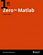Zero to MATLAB (Adam L. Lambert, PhD)

The book is structured as a tutorial rather than a reference. The MATLAB documentation is extensive and so my focus will be to outline basic algorithmic thinking and MATLAB syntax with explicit examples.

•Scientific Computing with MATLAB (Paul Gribble)

The goal of this book is to provide you with skills in scientific computing: tools and techniques that you can use in your own scientific research. We will focus on learning to think about experiments and data in a computational framework.

•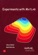Experiments with MATLAB (Cleve Moler)

This book provides an excellent initiation into programming in MATLAB while serving as a teaser for more advanced topics. It provides a structured entry into MATLAB programming through well designed exercises.

•Numerical Computing with MATLAB (Cleve B. Moler)

This book is a lively textbook for an introductory course in numerical methods, MATLAB, and technical computing. It helps readers learn enough about the mathematical functions in MATLAB to use them correctly, and modify them appropriately, etc.

•Numerical Methods and MATLAB Programming for Engineers

It covers the fundamentals while emphasizing the most essential numerical methods. Readers will enhance their programming skills using MATLAB to implement algorithms, discover how to use MATLAB to solve problems in science and engineering.

•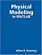Physical Modeling in MATLAB (Allen B. Downey)

Written for beginners, this book provides an introduction to programming in MATLAB and simulation of physical systems. you will learn some programming, some modeling, and some simulation.

•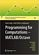Programming for Computations - MATLAB/Octave (Svein Linge)

This book presents computer programming as a key method for solving mathematical problems using MATLAB and Octave. It is intended for novice programmers and engineers. Each treated concept is illustrated and explained in detail by means of working examples.

•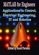MATLAB for Engineers: Applications in Control, EE, IT and Robotics

The book presents several approaches in the key areas of practice for which the MATLAB software package was used. Topics covered include applications for: Motors, Power systems , Robots, Vehicles.

•MATLAB Succinctly (Dmitri Nesteruk)

Learn the essential skills needed to use the flexible MATLAB system. You will be able to apply the highly modular system towards the purposes you need by harnessing the power of its different toolboxes.

•Applications from Engineering with MATLAB Concepts

This book presents a collection of MATLAB-based chapters of various engineering background. Instead of giving exhausting amount of technical details, authors were rather advised to explain relations of their problems to actual MATLAB concepts.

•MATLAB Applications for the Practical Engineer (Kelly Bennett)

This book is a collection of diverse applications of MATLAB. Each chapter presents a novel application and use of MATLAB for a specific result. MATLAB isn't everything to a practicing engineer, its just most everything.

•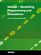MATLAB - Modelling, Programming and Simulations (E. P. Leite)

This is an authoritative guide to generating readable, compact, and verifiably correct MATLAB programs. It is ideal for undergraduate engineering courses in Mechanical, Aeronautical, Civil, and Electrical engineering that require/use MATLAB.

•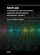MATLAB: Tool for Scientific Computing and Engineering Apps, Vol 1

This is the 1st book in a three-volume series deploying MATLAB-based applications in almost every branch of science. This volume covers topics from different areas of engineering, signal and image processing based on the MATLAB environment.

•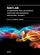MATLAB: Tool for Scientific Computing and Engineering Apps, Vol 2

This is the 2nd book in a three-volume series. This volumehe covers topics electronic engineering and computer science, MATLAB/SIMULINK as a tool for engineering applications, and telecommunication and communication systems.

•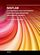MATLAB: Tool for Scientific Computing and Engineering Apps, Vol 3

This is the 3rd book in a three-volume series. This volume consists of mathematical methods in the applied sciences by using MATLAB, MATLAB applications of general interest, and MATLAB for educational purposes.

•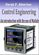Control Engineering: An Introduction with the Use of MATLAB

The book covers the basic aspects of linear single loop feedback control theory. Explanations of the mathematical concepts used in classical control such as root loci, frequency response and stability methods are explained by making use of MATLAB.

•MATLAB - A Ubiquitous Tool for the Practical Engineer (C. Ionescu)

This book is for both students and engineers with wide range of applications in which MATLAB is the working framework, shows that it is a powerful, comprehensive and easy-to-use environment for performing technical computations.

•Engineering Education and Research Using MATLAB (Ali H. Assi)

This book presents research works using MATLAB tools, including techniques for programming and developing GUI, dynamic systems, electric machines, signal and image processing, power electronics, mixed signal circuits, genetic programming, etc.

•Applications of MATLAB in Science and Engineering (Michalowski)

The book illustrates a wide range of areas where MATLAB tools are applied, including mathematics, physics, chemistry, mechanical engineering, biological and medical sciences, communication and control systems, digital signal, etc.

•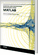Scientific and Engineering Applications Using MATLAB (E. P. Leite)

The purpose of this book is to present 10 scientific and engineering works whose numerical and graphical analysis were all constructed using the power of MATLAB tools.

•Neural Network Toolbox for MATLAB (Howard Demuth, et al)

It provides algorithms, functions, and apps to create, train, visualize, and simulate neural networks. You can perform classification, regression, clustering, dimensionality reduction, time-series forecasting, and dynamic system modeling and control.

•Biological Signal Analysis with MATLAB (R. Palaniappan)

This book will provide the reader with an understanding of biological signals and digital signal analysis techniques such as conditioning, filtering, classification and statistical validation for solving practical biological signal analysis problems using MATLAB.

Book Categories
 :All CategoriesRecent BooksMiscellaneous BooksComputer LanguagesComputer ScienceData Science/DatabasesElectrical EngineeringJava and Java EE (J2EE)Linux and UnixMathematicsMicrosoft and .NETMobile ComputingNetworking and CommunicationsSoftware EngineeringSpecial TopicsWeb Programming
Other Categories